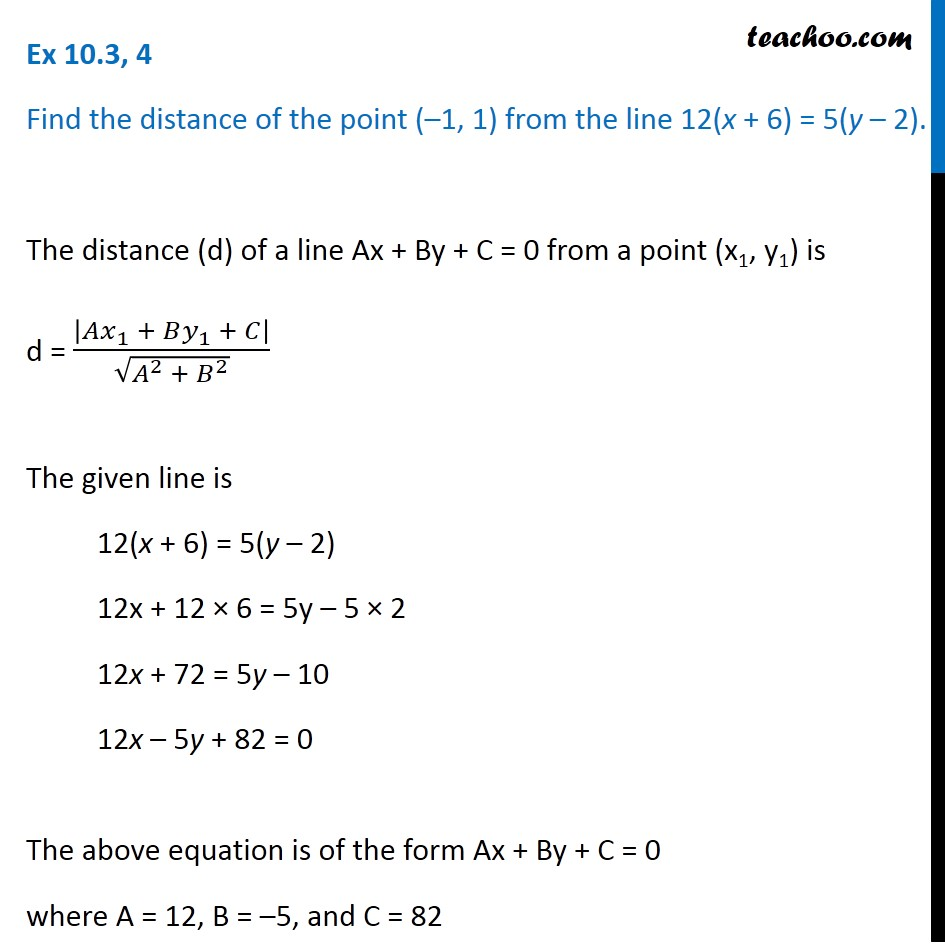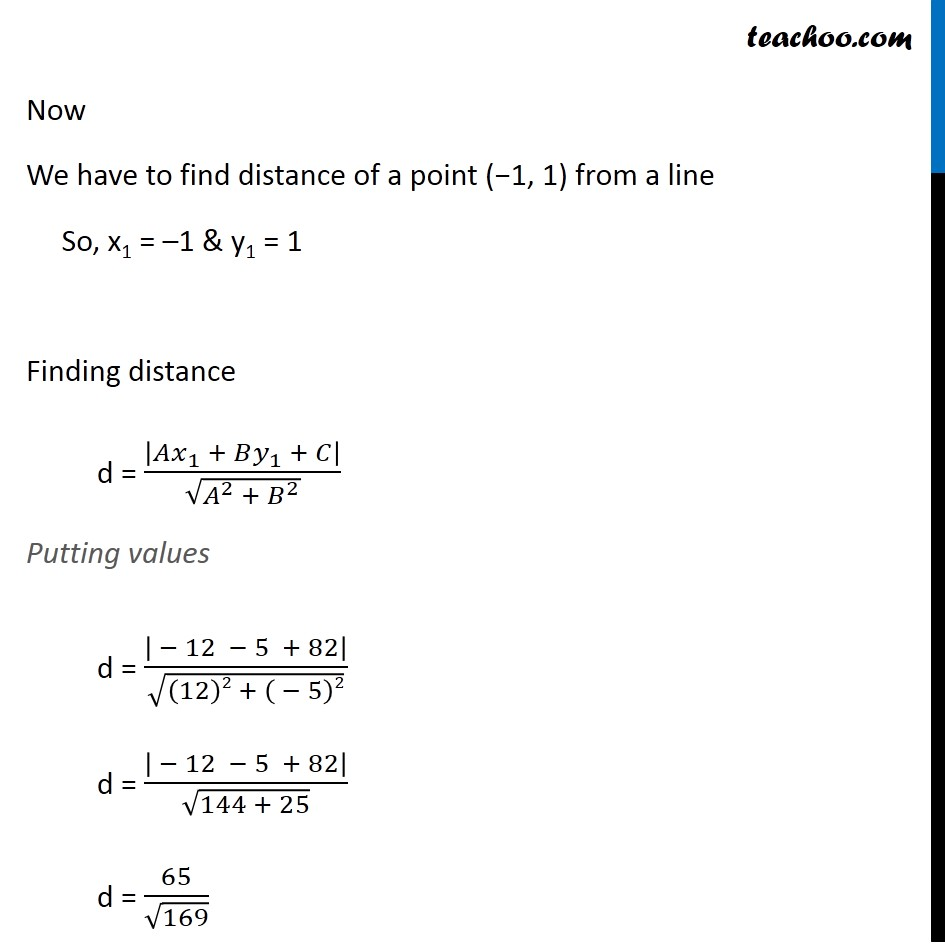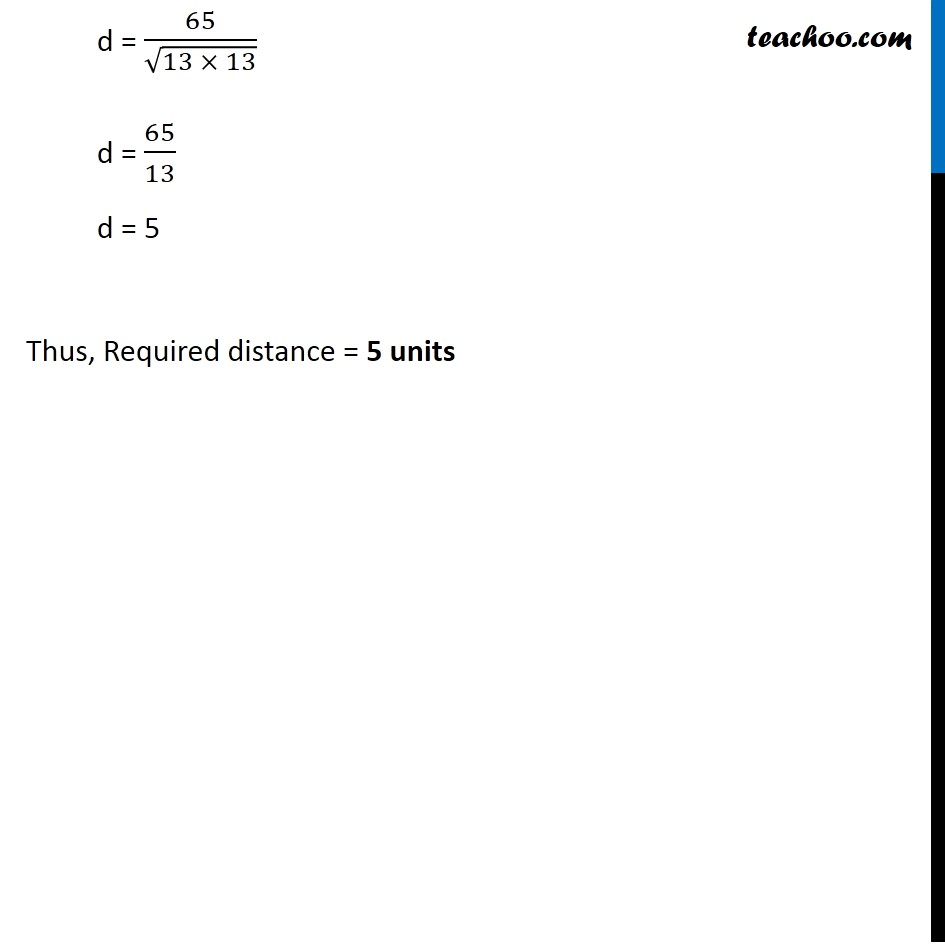Ex 9.3

Chapter 9 Class 11 Straight Lines
Serial order wiseLearn in your speed, with individual attention - Teachoo Maths 1-on-1 Class

### Transcript

Ex 9.3, 3 Find the distance of the point (–1, 1) from the line 12(x + 6) = 5(y – 2). The distance (d) of a line Ax + By + C = 0 from a point (x1, y1) is d = |𝐴𝑥_1 + 𝐵𝑦_1 + 𝐶|/√(𝐴^2 + 𝐵^2 ) The given line is 12(x + 6) = 5(y – 2) 12x + 12 × 6 = 5y – 5 × 2 12x + 72 = 5y – 10 12x – 5y + 82 = 0 The above equation is of the form Ax + By + C = 0 where A = 12, B = –5, and C = 82 Now We have to find distance of a point (−1, 1) from a line So, x1 = –1 & y1 = 1 Finding distance d = |𝐴𝑥_1 + 𝐵𝑦_1 + 𝐶|/√(𝐴^2 + 𝐵^2 ) Putting values d = | − 12 − 5 + 82|/√((12)2 + ( − 5)2) d = | − 12 − 5 + 82|/√(144 + 25) d = 65/√169 d = 65/√(13 × 13) d = 65/13 d = 5 Thus, Required distance = 5 units